JMSLTM Numerical Library 7.2.0
com.imsl.math

Class NumericalDerivatives

• All Implemented Interfaces:
Serializable, Cloneable

public class NumericalDerivatives
extends Object
implements Serializable, Cloneable
Compute the Jacobian matrix for a function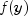with m components in n independent variables.

NumericalDerivatives uses divided finite differences to compute the Jacobian. This class is designed for use in numerical methods for solving nonlinear problems where a Jacobian is evaluated repeatedly at neighboring arguments. For example this occurs in a Gauss-Newton method for solving non-linear least squares problems or a non-linear optimization method.

NumericalDerivatives is suited for applications where the Jacobian is a dense matrix. All cases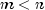,, or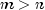are allowed. Both one-sided and central divided differences can be used.

The design allows for computation of derivatives in a variety of contexts. Note that a gradient should be considered as the special case with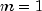,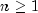. A derivative of a single function of one variable is the case,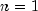. Any non-linear solving routine that optionally requests a Jacobian or gradient can use NumericalDerivatives. This should be considered if there are special properties or scaling issues associated with. Use the method setDifferencingMethods to specify different differencing options for numerical differentiation. These can be combined with some analytic subexpressions or other known relationships.

The divided differences are computed using values of the independent variables at the initial point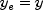, and differenced points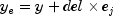. Here the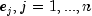, are the unit coordinate vectors. The value for each difference del depends on the variable j, the differencing method, and the scaling for that variable. This difference is computed internally. See setPercentageFactor for computational details. The evaluation of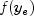is normally done by the user-provided method NumericalDerivatives.Function.f, using the values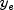. The index j and valuesare arguments to NumericalDerivatives.Function.f.

The computational kernel of evaluateJ performs the following steps:

1. evaluate the equations at the point y using NumericalDerivatives.Function.f.
2. compute the Jacobian.
3. compute the difference at.

By default, evaluateJ uses NumericalDerivatives.Function.f in step 3. The user may choose to override the evaluateF method to extend the capability of the class beyond the default.

There are six examples provided which illustrate various ways to use NumericalDerivatives. A discussion of the expected errors for these difference methods is found in A First Course in Numerical Analysis, Anthony Ralston, McGraw-Hill, NY, (1965).

Example: One-Sided Differences, Example: Skipping A Gradient Component, Example: Accumulation Of A Component, Example: Central Differences, Example: Hessian Approximation, Example: Usage With Class MinUnconMultiVar , Serialized Form
• Field Summary

Fields
Modifier and Type Field and Description
static int ACCUMULATE
Indicates the accumulation of the result from whatever type of differences have been specified previously into initial values of the Jacobian.
static int CENTRAL
Indicates central differences.
static int ONE_SIDED
Indicates one sided differences.
static int SKIP
Indicates a variable to be skipped.
• Method Summary

Methods
Modifier and Type Method and Description
protected double[] evaluateF(int varIndex, double[] y)
This method is provided by the user to compute the function values at the current independent variable values y.
double[][] evaluateJ(double[] y)
Evaluates the Jacobian for a system of (m) equations in (n) variables.
double[] getPercentageFactor()
Returns the percentage factor for differencing.
double[] getScalingFactors()
Returns the scaling factors for the y values.
int[] getStatus()
Returns status information.
void setDifferencingMethods(int[] options)
Sets the methods used to compute the derivatives
void setInitialF(double[] valueF)
Set the initial function values.
void setPercentageFactor(double[] factor)
Sets the percentage factor for differencing
void setScalingFactors(double[] scale)
Sets the scaling factors for the y values.
• Field Detail

• ACCUMULATE

public static final int ACCUMULATE
Indicates the accumulation of the result from whatever type of differences have been specified previously into initial values of the Jacobian.
Constant Field Values
• CENTRAL

public static final int CENTRAL
Indicates central differences.
Constant Field Values
• ONE_SIDED

public static final int ONE_SIDED
Indicates one sided differences.
Constant Field Values
• SKIP

public static final int SKIP
Indicates a variable to be skipped.
Constant Field Values
• Constructor Detail

• NumericalDerivatives

public NumericalDerivatives(NumericalDerivatives.Function fcn)
Constructor for NumericalDerivatives.
Parameters:
fcn - a Function object which is a user-supplied function to evaluate the equations at the point y.
• Method Detail

• evaluateF

protected double[] evaluateF(int varIndex,
double[] y)
This method is provided by the user to compute the function values at the current independent variable values y. If the user does not override the evaluateF method, then NumericalDerivatives.Function.f is used to compute the function values.
Parameters:
varIndex - an int which indicates the index of the variable to perturb.
y - a double array of length n, the point at which the function is to be evaluated.
Returns:
a double array of length m. The equations evaluated at the point y.
• evaluateJ

public double[][] evaluateJ(double[] y)
Evaluates the Jacobian for a system of (m) equations in (n) variables.
Parameters:
y - a double array of length n, the point at which the Jacobian is to be evaluated.
Returns:
a double matrix containing the Jacobian. Columns that are accumulated must have the additive term defined on entry or else be set to zero. Columns that are skipped can be defined either before or after the evaluateJ method is invoked.
• getPercentageFactor

public double[] getPercentageFactor()
Returns the percentage factor for differencing.
Returns:
a double array containing the percentage factor for differencing. See setPercentageFactor for more detail.
• getScalingFactors

public double[] getScalingFactors()
Returns the scaling factors for the y values.
Returns:
a double array containing the scaling factors.
• getStatus

public int[] getStatus()
Returns status information. This information might prove useful to the user wanting to gain better control over the differencing parameters. This information can often be ignored.
Returns:
an int array containing the ten diagnostic values described in the following table. These values can be used to monitor the progress or expense of the Jacobian computation.  index Description 0 the number of times a function evaluation was computed. 1 the number of columns in which three attempts were made to increase a percentage factor for differencing (i.e. a component in the factor array) but the computed del remained unacceptably small relative to y[j-1] or scale[j-1]. In such cases the percentage factor is set to 1.4901161193847656e-8, which is the square root of machine precision 2 the number of columns in which the computed del was zero to machine precision because y[j-1] or scale[j-1] was zero. In such cases del is set to 1.4901161193847656e-8, which is the square root of machine precision 3 the number of Jacobian columns which had to be recomputed because the largest difference formed in the column was close to zero relative to scale, where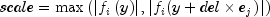and i denotes the row index of the largest difference in the column currently being processed. index = 9 gives the last column where this occurred. 4 the number of columns whose largest difference is close to zero relative to scale after the column has been recomputed. 5 the number of times scale information was not available for use in the roundoff and truncation error tests. This occurs when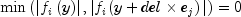where i is the index of the largest difference for the column currently being processed. 6 the number of times the increment for differencing (del) was computed and had to be increased because (scale[j-1]+del) - scale[j-1]) was too small relative to y[j-1] or scale[j-1]. 7 the number of times a component of the factor array was reduced because changes in function values were large and excess truncation error was suspected. index = 8 gives the last column in which this occurred. 8 the index of the last column where the corresponding component of the factor array had to be reduced because excessive truncation error was suspected. 9 the index of the last column where the difference was small and the column had to be recomputed with an adjusted increment (see index = 3). The largest derivative in this column may be inaccurate due to excessive roundoff error.
• setDifferencingMethods

public void setDifferencingMethods(int[] options)
Sets the methods used to compute the derivatives
Parameters:
options - an int array of length n, containing the methods used to compute the derivatives. options[i] is the method to be used for the i-th variable. options[i] can be one of the values in the table which follows. The default is to use ONE_SIDED differences for each variable.  Entry Description ONE_SIDED Indicates one sided differences. CENTRAL Indicates central differences. ACCUMULATE Indicates the accumulation of the result from whatever type of differences have been specified previously into initial values of the Jacobian. SKIP Indicates a variable to be skipped.
• setInitialF

public void setInitialF(double[] valueF)
Set the initial function values. Use the values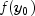, where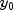is the initial value of the independent variables located in array y.
Parameters:
valueF - a double array of length m containing the initial function values,. Default: all values are 0.0.
• setPercentageFactor

public void setPercentageFactor(double[] factor)
Sets the percentage factor for differencing

For each divided difference for variable j the increment used is del. The value of del is computed as follows: First define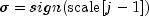. If the user has set the elements of array scale to non-default values, then define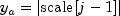. Otherwise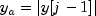and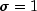. Finally compute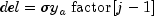. By changing the sign of scale[j-1], the difference del can have any desired orientation, such as staying within bounds on variable j. For central differences, a reduced factor is used for del that normally results in relative errors as small as machine precision to the 2/3 power.

Parameters:
factor - a double array of length n containing the percentage factor for differencing. Except for initialization, the factor array should not be altered in the evaluateF method. The elements of factor must be such that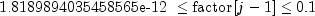where 1.8189894035458565e-12 is machine precision to the three-fourths power.
Default: all elements of factor are set to 1.4901161193847656e-8, which is the square root of machine precision.
• setScalingFactors

public void setScalingFactors(double[] scale)
Sets the scaling factors for the y values. The user can also use scale to provide appropriate signs for the increments.
Parameters:
scale - a double array of length n containing the scaling factors. Default: all values are 1.0.
JMSLTM Numerical Library 7.2.0